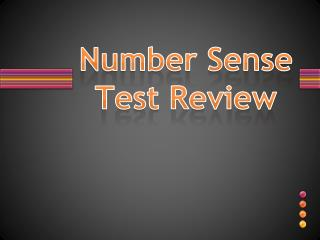DownloadDownload PresentationNumber Sense Test Review

# Number Sense Test Review

Download Presentation## Number Sense Test Review

- - - - - - - - - - - - - - - - - - - - - - - - - - - E N D - - - - - - - - - - - - - - - - - - - - - - - - - - -
##### Presentation Transcript

1. Number Sense Test Review

3. Find the GCF of 20 and 24

4. Find the GCF of25 , 125, and 200

5. Find the GCF! 30 53

6. Relatively PrimeGCF = 1

7. Find the GCf of 9, 36, 60

8. 3

9. Find the LCM of 18 and 30

10. 90

11. Find the LCM! 18, 20, 24

12. LCM = 360

13. Find the LCM 9 and 16

14. Relatively PrimeLCM = 144

15. N = 23, Commutative Property of +

16. N = 3, Distributive Property

17. Use variables a,b and c to define the Associative Property of Multiplication.

18. a(bc) = (ab)c

19. a (b + c) = ab + ac

20. Find the GCF and LCM of 12 and 20

21. GCF = 4 LCM = 60

22. Find the GCF and LCM of 9, 12, and 25

23. ** GCF = 1 ** LCM = 900

24. GCF or LCM ? You decide. Two bikers are riding a circular path. The first rider completes a round in 12 minutes. The second rider completes a round in 18 minutes. If they both started at the same place and time and go in the same direction, after how many minutes will they meet again at the starting point?

25. LCM

26. GCF or LCM ? You decide. Z100 gave away a Z \$100 bill for every 100th caller. Every 30th caller received free concert tickets. How many callers must get through before one of them receives both a coupon and a concert ticket?

27. LCM LCM

28. GCF or LCM ? You decide. Mrs. Evans has 120 crayons and 30 pieces of paper to give to her students. What is the largest number of students she can have in her class so that each student gets an equal number of crayons and equal amount of paper?

29. ag GCF

30. -8 + 24

31. 16

32. -12 – 30

33. -42

34. -7  4

35. -28

36. 32 (-4)

37. -8

38. -45 + 30

39. -15

40. -12 – (-15)

41. 3

42. 12 + c = -18

43. C = -30

44. -5x = 30